###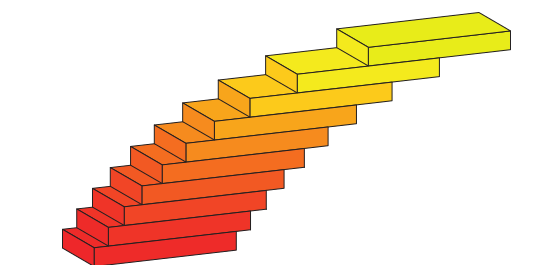STACK

STACK is an assessment system for mathematics, science and related disciplines, designed to enable students to answer questions with a mathematical expression, such as Arithmetic expressions, Fractional expressions, Algebraic expression and much more.

• Students type in mathematical expressions, and are not restricted to multiple choice.
• Separate validation and assessment with an emphasis on formative assessment.
• Random generation of questions.
• A wide variety of input types, including line by line working.
• Support for multipart questions.
• Support for scientific units and significant figures

## Equivalent answers of the right form

STACK can accept equivalent expressions, for example (x+1)2=x2+2x+1. STACK can also establish the form of an answer, for example if it is factorised. STACK is designed to let teachers specify independent properties required in an answer.

## Ask for examples

The system can mark questions that ask the student to provide an example.

Give an example of a function f(x) with a minimum at x=0 and a maximum at x=2. Rather than comparing the student's answer to the teacher's answer, STACK checks that the answer has the required properties. Giving examples is a higher-order skill that is impossible to assess with conventional Computer Aided Assessment (CAA) systems.

## Intelligent randomisation

Randomising questions is invaluable in ensuring students can practice and reducing sharing of answers. The trick is to reverse-engineer the randomised question from a randomised answer. Computer algebra is invaluable to support this process.

## Give feedback and partial credit

Consider a question like: Give an example of a cubic polynomial with the following properties:

• p(0)=1,
• p(x)=0 at x=2 and at x=3.

Here, STACK can check each condition separately on the student's answer and assign partial credit accordingly. If the student gave the answer p(x)=x2−5x+6 for example, then STACK could reply: Your answer does have zeros at the required points but its value at zero is not equal to 1. You received 2 out of 3 points. Please try again. There are a variety of ways in which feedback can be given, including plotting the students' answer against the teacher's answer.

## Multipart questions

STACK supports multipart questions, like the following:

(a) differentiate x2+5x with respect to x.

You can have follow-through marking for situations where, for example, the student enters a wrong expression to part (a), but correctly substitutes in values into their expression in part (b). Here, STACK can recognise the work the student put into part (b), even though their answer is different from the "correct" answer.

STACK quiz questions are available to add to your quiz activities within UR Courses.

1. Create a new quiz activity. If you aren't sure how to create a quiz activity please refer to adding a quiz to your course.

2. Once the quiz has been created,

• click the Edit quiz button,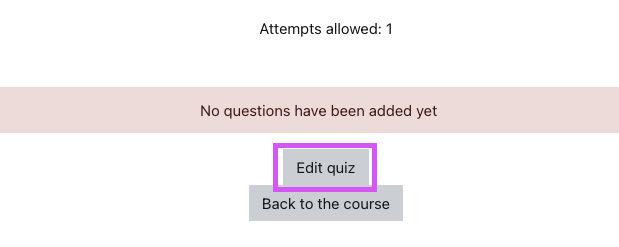• or click the action menu button, in the top right corner of the quiz, and click edit quiz button as highlighted on the image below.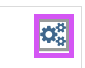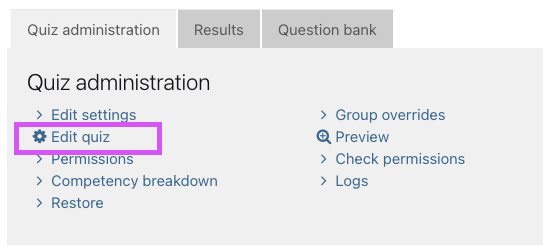• To add a question, click the add link button and then click Add new question.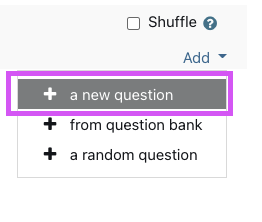• Choose question type popup window will open, scroll down to the bottom of the popup window and the stack question type should be presented as highlighted on the image below.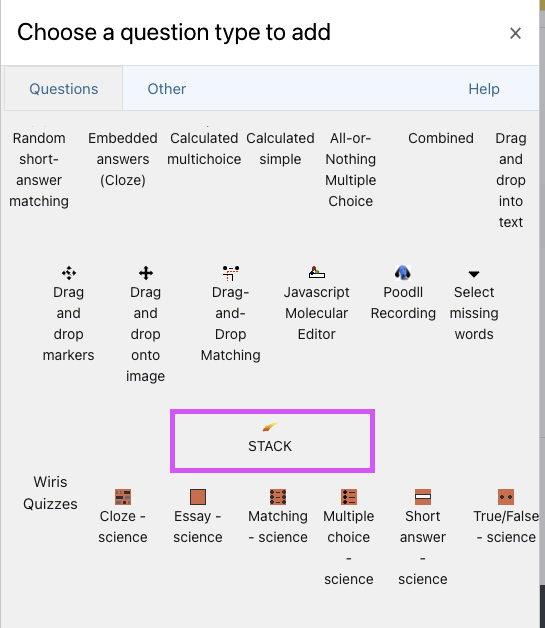• Please visit STACK Docs? A detailed documentation of STACK and resource.

### Question tips and reference

There are many ways to organize and setup STACK questions within your course. The following are some helpful video references created by the STACK project.

How to write quality STACK questions
How to make STACK questions look nice

### Project demo

The STACK project has a public demo which provides some example and sample STACK questions that can help get you started using STACK.

The rest of this course contains sample collections of quizzes on common topics, such as Calculus and Algebraic manipulation.

This course also contains a large number of additional questions which are not collected into quizzes.

### Example content of STACK Demo

• Learning to use STACK for students The following questions are designed to help students understand the STACK layout and syntax.
• Demonstration quiz
• Authoring features These quizzes demonstrate various authoring features in STACK.
• Sample collections
• Diagnostic tests In April 2010 the National HE STEM Programme funded a mini-project to take existing diagnostic tests in core mathematics and develop similar automatic tests for the STACK computer aided assessment system.

### Useful URL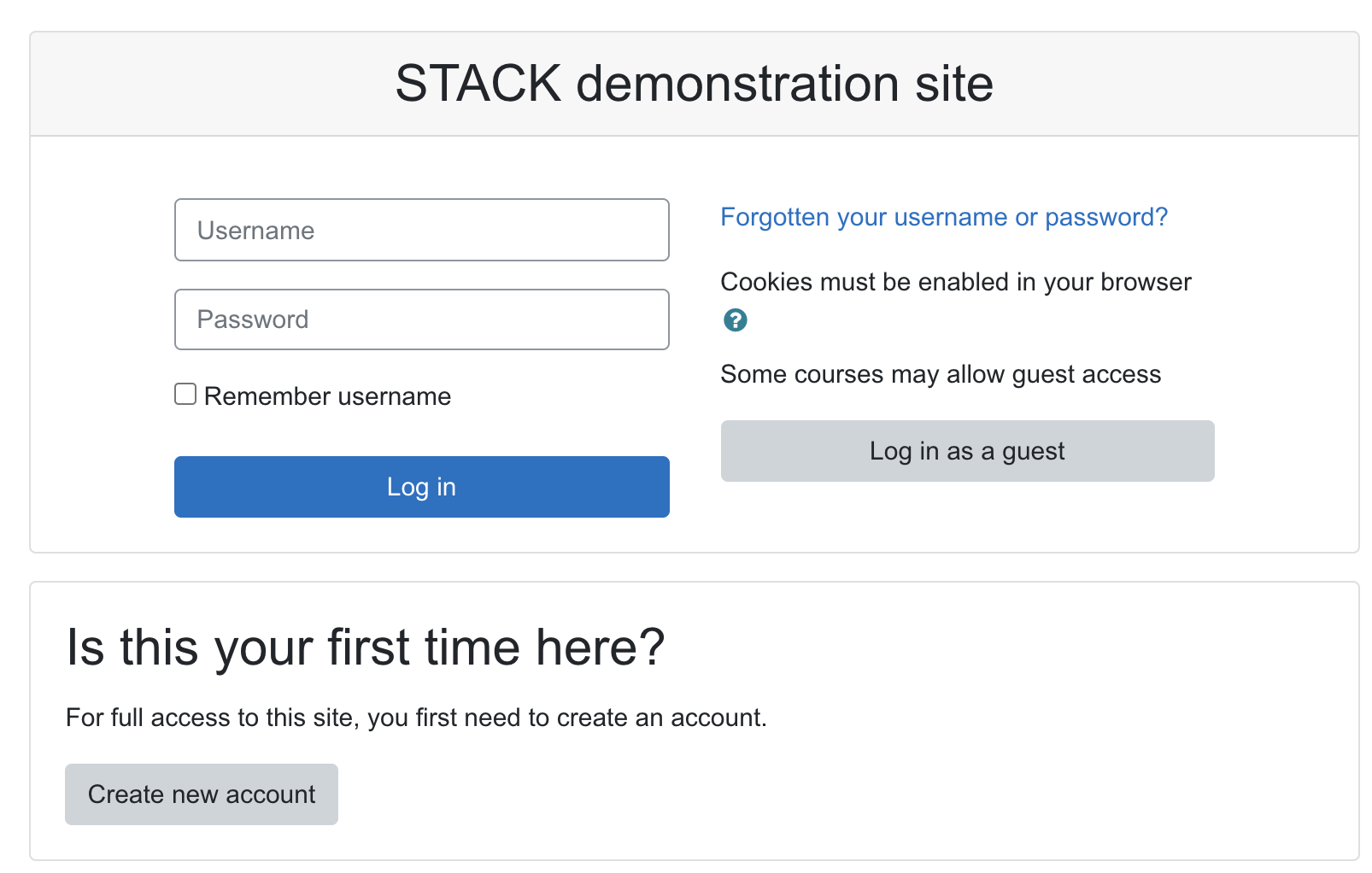To be able to access the content of this demo course page. You need to create an account signup here.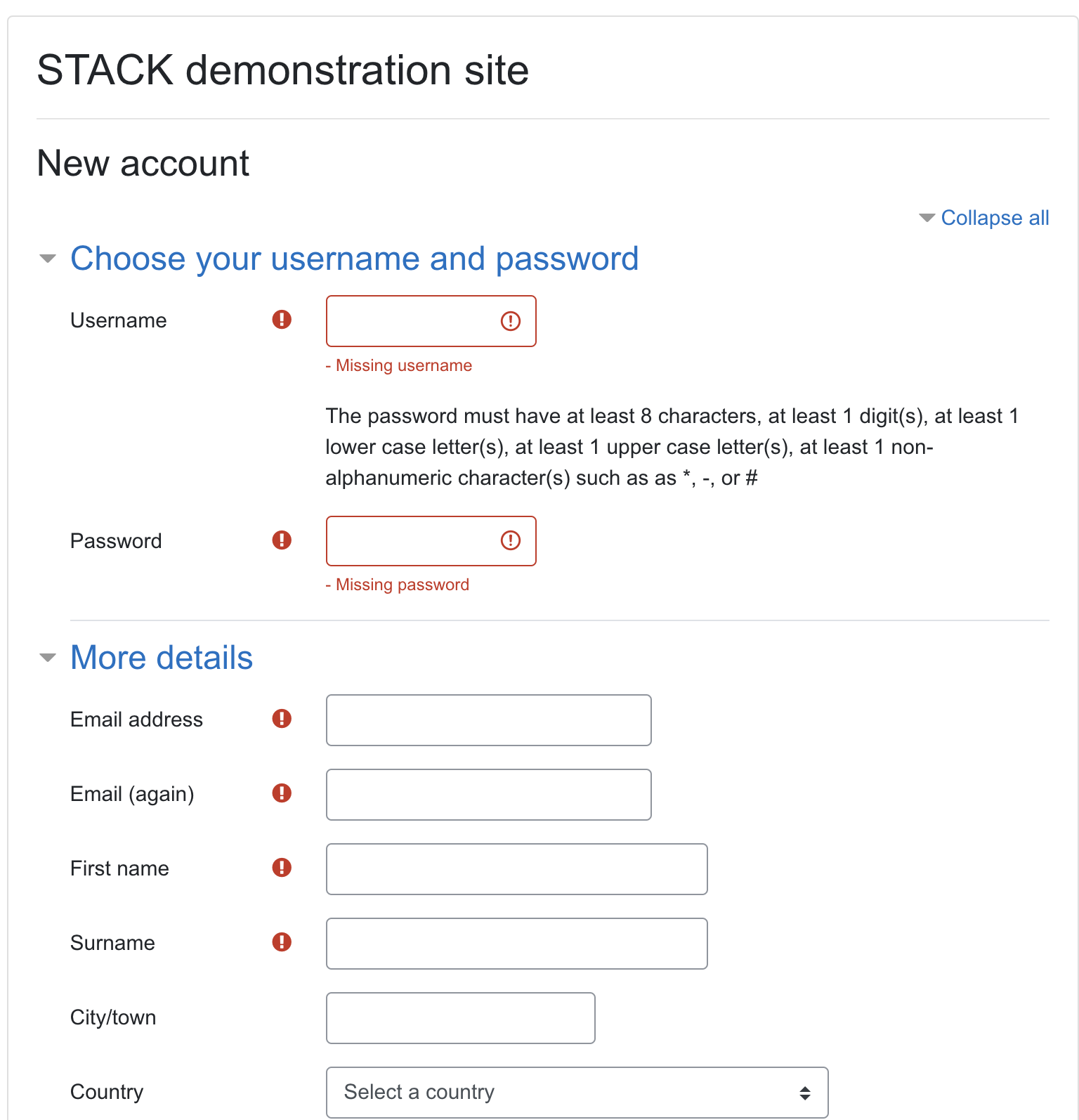### Quiz samples

Feel free to try out quiz samples that is available in UR Courses and you can copy this as well in github and import it via xml.

STACK-4.3.9-demo is available in UR Courses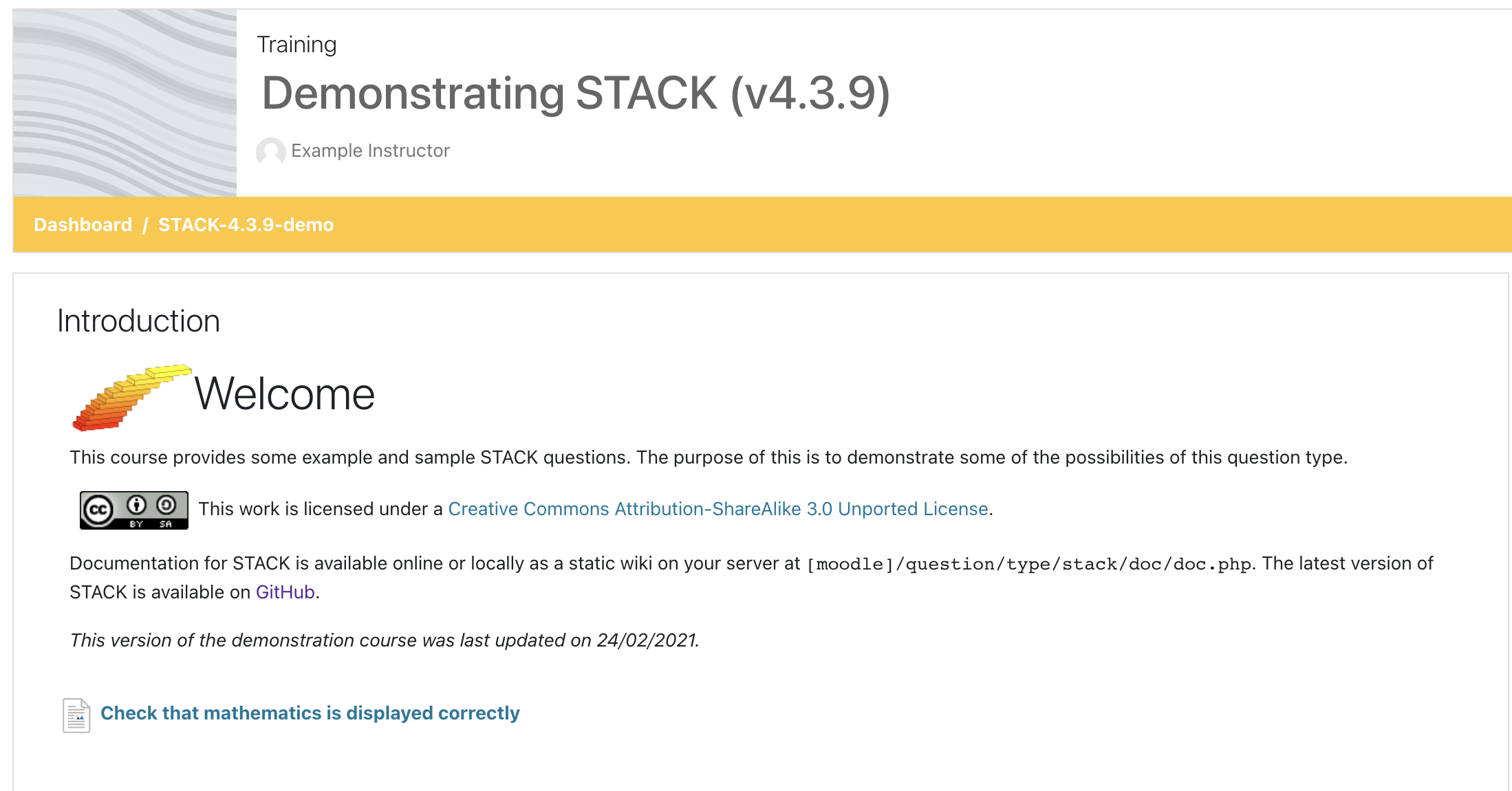Quiz Examples availble in Testing Course page.